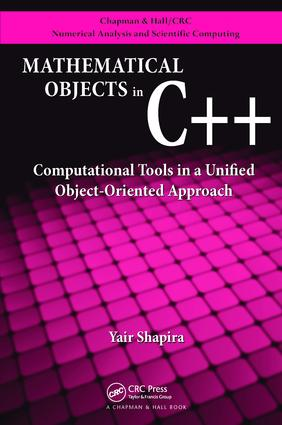# Mathematical Objects in C++

## Computational Tools in A Unified Object-Oriented Approach, 1st Edition

CRC Press

609 pages | 130 B/W Illus.

##### Purchasing Options:\$ = USD
Paperback: 9781138113763
pub: 2017-06-28
\$82.95
x
Hardback: 9781439811474
pub: 2009-06-19
Currently out of stock
\$220.00
x
eBook (VitalSource) : 9780429092497
pub: 2009-06-19
from \$39.98

FREE Standard Shipping!

### Description

Emphasizing the connection between mathematical objects and their practical C++ implementation, this book provides a comprehensive introduction to both the theory behind the objects and the C and C++ programming. Object-oriented implementation of three-dimensional meshes facilitates understanding of their mathematical nature. Requiring no prerequisites, the text covers discrete mathematics, data structures, and computational physics, including high-order discretization of nonlinear equations. Exercises and solutions make the book suitable for classroom use and a supporting website supplies downloadable code.

### Reviews

"This is an exciting book on mathematics on one hand and computer programming on the other, and the reader can concentrate on one of the topics or both. The mathematics part focuses on teaching mathematics through constructive algorithms, ready for computer implementation. I believe this is the future view of these classical mathematical subjects. Many will find this book a major refresher of the mathematics literature. The programming part of the book serves as a very good introduction to C and C++, using meaningful and motivating examples from mathematics. The book is full of useful exercises of various difficulty. This fact makes the book very suitable as a textbook for a mathematics or computer science course."

—Hans Petter Langtangen, Simula Research Laboratory and University of Oslo, Norway

NUMBERS

Natural Numbers

Integer Numbers

Rational Numbers

Real Numbers

Complex Numbers

GEOMETRICAL OBJECTS

Euclidean Geometry

Analytic Geometry

COMPOSITE MATHEMATICAL OBJECTS

Sets

Vectors and Matrices

Multilevel Objects

Graphs

Polynomials

INTRODUCTION TO C

Basics of Programming

Recursion

INTRODUCTION TO C++

Objects

Vectors and Matrices

Dynamic Vectors and Lists

IMPLEMENTATION OF COMPUTATIONAL OBJECTS

Trees

Graphs

Sparse Matrices

Meshes

Triangulation

THREE-DIMENSIONAL APPLICATIONS

Mesh of Tetrahedra

Polynomials

Sparse Polynomials

Stiffness and Mass Matrices

Splines

Appendix: Solutions of Exercises

References

Index

Exercises appear at the end of each chapter.

### About the Author

Yair Shapira is in the computer science department at Technion. His research interests include multigrid, preconditioning, and numerical methods.

### Subject Categories

##### BISAC Subject Codes/Headings:
COM051010
COMPUTERS / Programming Languages / General
MAT000000
MATHEMATICS / General
MAT021000
MATHEMATICS / Number Systems